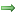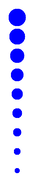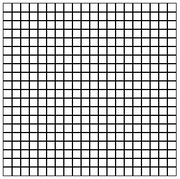# Lab Exercise for Assignment 4

## COMP102/112 2022 Tri 1: Lab Exercise 4

#### Goals

This assignment will give you practice at writing programs that:
• use conditionals
• use for loop (foreach loops and for loops)
• have methods that return values

#### Preparation

Download the zip file for lab exercise 4 and extract it to the `COMP-102-112-2022T1-Lab4` folder in your home folder. It should contain templates for the exercises program. Read through the exercises and run or view the demo so that you know what you need to do.

Later when the model answer to assignment 3 becomes available, make sure you understand all the components of the programs.

Also, go over the code examples from the lectures that used for loops.

#### Summary

• Foreach loops exercise:Write little programs that have foreach loop.
• For loops exercise:Write little programs that have loops and nested loops.

### Exercise Series 1: Programming with foreach loops

For the first series of exercises, you must complete methods that use foreach loops to repeat actions.

• If you are using a computer in a lab, you can run the `ForeachLoopsExercise` demo to see what your program should do (look in the `assig4-demos` folder).
• Or, you can watch the demo video.

Complete the following methods in the `ForeachLoopsExercise.java` file.

• `squareNumbers()` should read a sequence of numbers, using `UI.askNumbers()`, then print out the squares of each number.
For example, if they enter
`4 21 20 9 12 done`
it should print
`16 441 400 81 144`
(either on one line or on separate lines). If you select "Set Input" on the UI frame's MENU then choose the file `numbers.txt`, the UI will automatically input the data from that file. This may help with testing your program.

• `addBigNumbers()` should read a sequence of numbers, using `UI.askNumbers()`, then print the sum of all the numbers that are bigger than 9.
For example, if they enter
`4 21 20 9 12 done`
it should add up 21+20+12 and print
`53`.
You can use the file `numbers.txt` to input data automatically.

• `printTHWords()` should read a sequence of words, using `UI.askStrings()`, then print out all the words containing "th".
For example, if they enter
`Mathilde is the happiest person other than Matthew`
it should print out
`Mathilde the other than Matthew`.
You can use the file `words.txt` to input data automatically.

• `drawDots()` should first read a sequence of numbers using `UI.askNumbers()`. Then, for each number n, if it is less than 300, it should draw a red dot (10 pixels diameter) at position (n, n). You can use the file `for-drawing-dots.txt` to input data automatically.

### Exercise Series 2: Programming with for loops

For the second series of exercises, you must complete methods that use for loops to repeat actions.

• If you are using a computer in a lab, you can run the `LoopsExercise` demo to see what your program should do (look in the `assig4-demos` folder).
• Or, you can watch the demo video.

Complete the following methods in the `LoopsExercise.java` file.

• `drawBubbles()` should draw a sequence of blue bubbles going up, getting bigger as they go. The smallest bubble should be 10 units in diameter. The rest of the bubbles should each be 4 units larger than above the previous bubble, and the center of each bubble should be BUBBLE_STEP units above the center of the previous bubble• `drawGridBoard()` should draw the the basic pattern for many board games.You should draw this as a grid made of lots of squares. Note that it should need nested loops.

• `printStars()` should print a triangular pattern of asterisks (*'s) - if size is 5, it should print 5 rows, with 1 asterisk in the first row, increasing up to 5 asterisks in the 5th row; if the size is 100, there should be 100 rows.
```*
**
***
****
*****
```